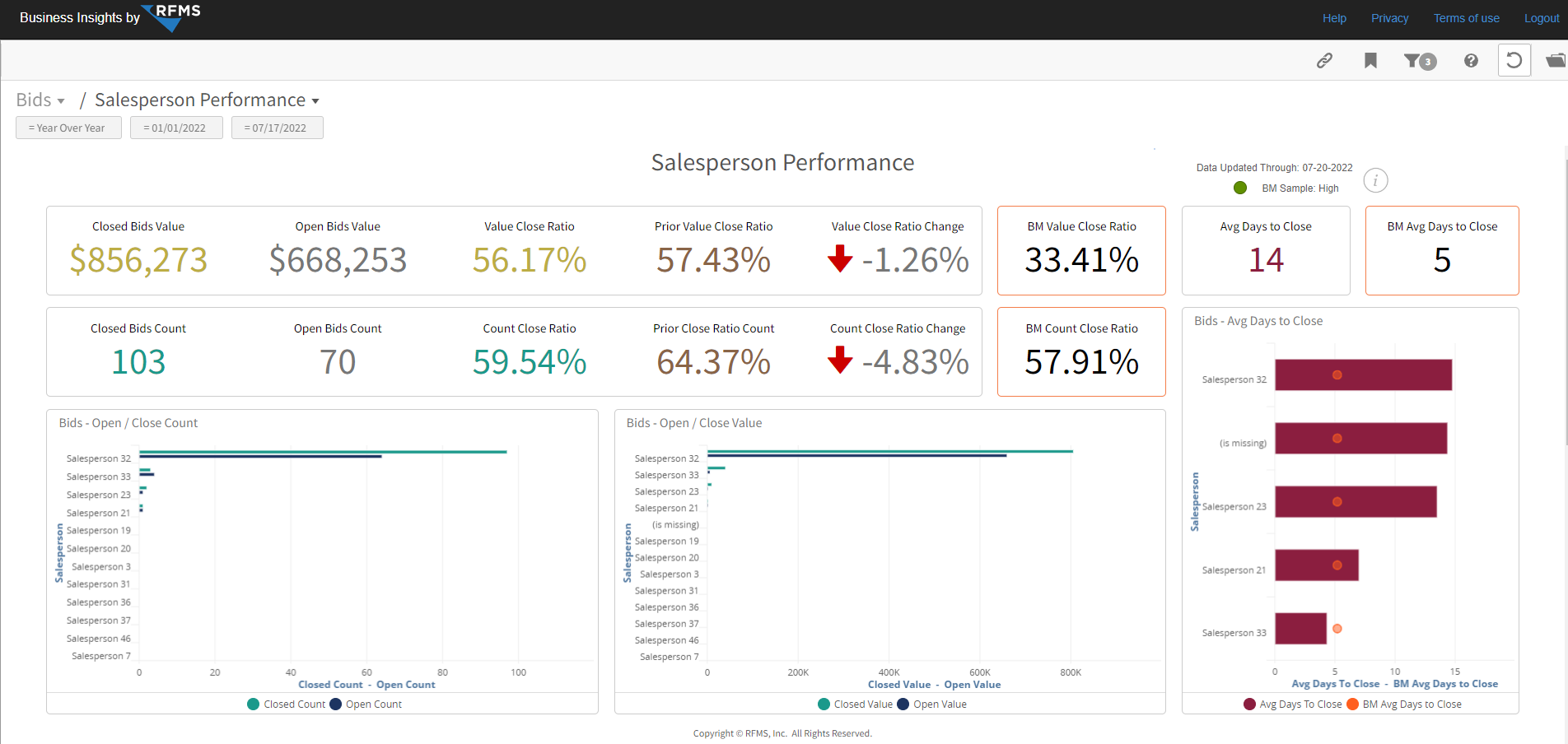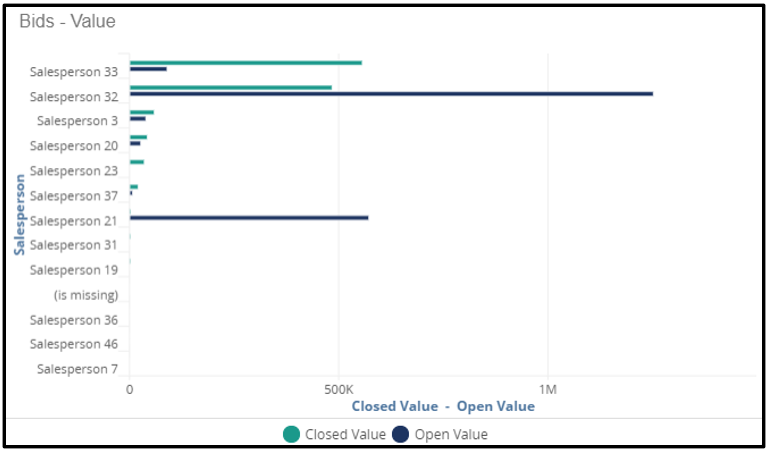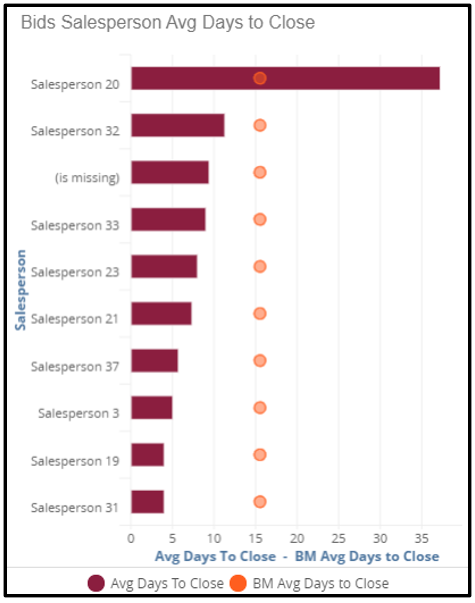# Bids Salesperson Performance

This dashboard displays the Salesperson performance for bids by count, value and avg days to close. Use the "My Stores", “My Salesperson”, "My Job Types" and multiple benchmark filters to further refine the data you want to display.Key Performance Indicator's (KPI's)

Closed Bid Value: The value of all closed bids for the active filters.

Open Bid Value: The value of all open bids for the active filters.

Value Close Ratio: The percentage of existing bids that are closed for the selected filters. This is calculated by dividing closed bid Value by the total bid value.

Prior Value Close Ratio: The percentage of existing bids that are closed for the prior period of the selected date range (Year over Year or Trailing Months) for all active filters.This value is calculated by dividing prior period closed bid value by prior period open bid value.

Value Close Ratio Change: The comparison of the prior period close ratio to the current close ratio. This is calculated by subtracting the prior value close ratio from the value close ratio for the selected date range.

BM Value Close Ratio: The percentage of existing bids that are closed for the selected date range for all qualified participating dealers. This is calculated by dividing all the Closed Bid Values by all the Open Bid Values.

Avg Days to Close: The total avg days to convert bids into orders for the selected date range.

BM Avg Days to Close:  Total avg days to close bids the selected date range for all qualified Business Insights subscribers.

Closed Bids Count: The number of all closed bids for the selected date range.

Open Bids Count: The number of all open bids for the selected date range.

Count Close Ratio: The percentage of closed bids divided by the total number of bids for the selected filters.

Prior Close Ratio Count: The percentage of closed bids.This is calculated by dividing the number of closed bids by the total number of bids for the prior period and selected filters.

Count Close Ratio Change: The difference between count close ratio and the prior close ratio count.

BM Count Close Ratio: The percentage of total number of closed bids divided by the total number of bids for qualified participating dealers for the active filters.

BM Avg Days to Close: Average number of days to close bids for all qualified participating dealers.

Charts

Bids - Count: The number of closed and open bids for each saleperson for all active filters.Bids - Value: The value of closed and open bids for each salesperson for the active filters.Avg Days To Close: The average days to close a bid versus benchmark value for each salesperson for the selected filters.